# 信号在脑子里面应该是什么样的（二）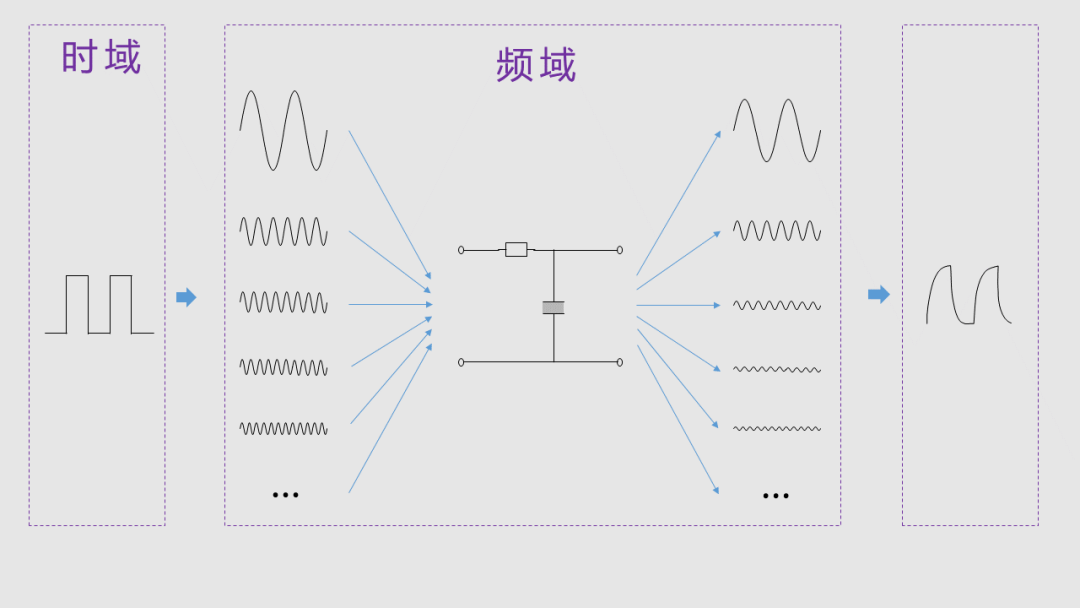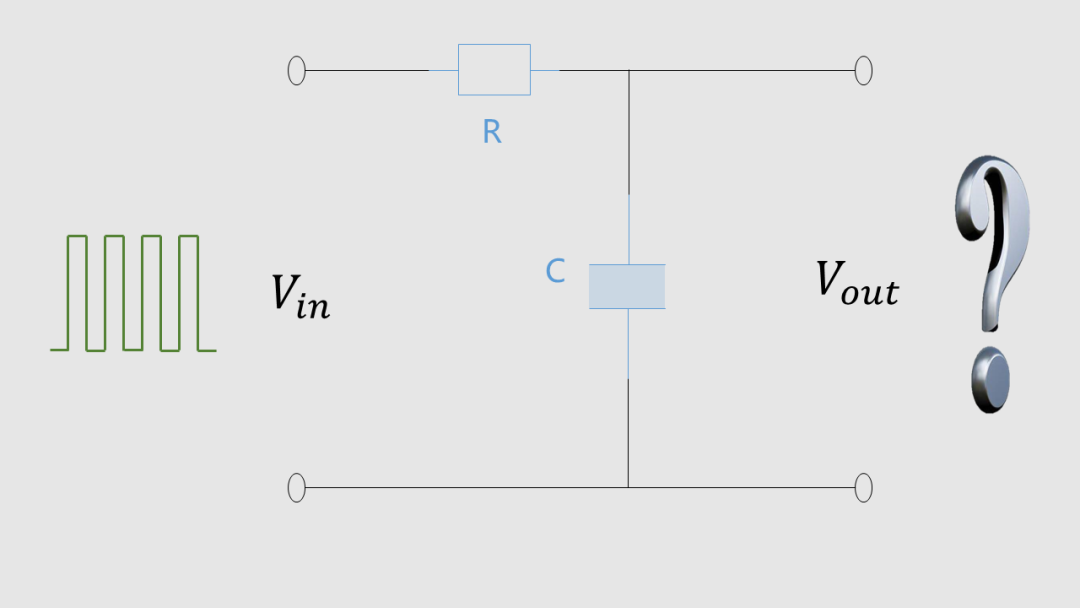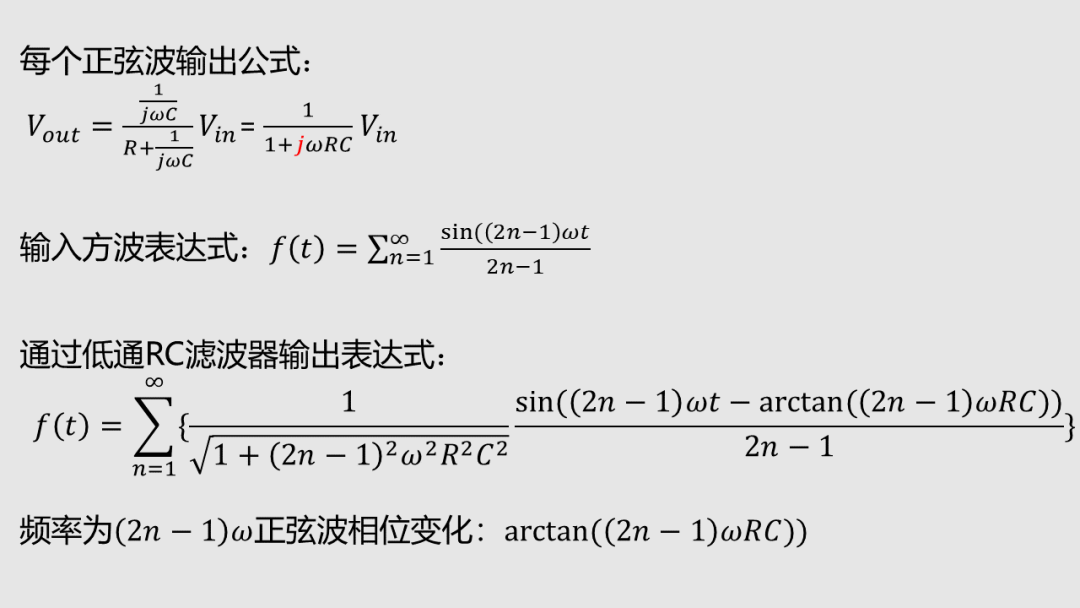Matlab代码：

``````%参数设置
R=1000/(2*pi);  %电阻值，2*pi只是为了方便是截止频率为整数
C=0.000001; %电容
Fc=1/(2*pi*R*C); %RC滤波器的截止频率
f=1;  %方波频率1Hz
omega=2*pi*f; %基频角频率w

%计算
t=-1:0.001:1 %坐标轴从-1到1，分辨率为0.001
n=[1:2:201];  %表示201谐波数的叠加   单行矩阵：1,3,5,7,9...201
An=4./(pi*n); %方波的各个谐波的系数  单行矩阵：1,1/3,1/5,1/7,1/9...1/201
Bn=sin(omega*n'*t); %方波的各个谐波分量  单列矩阵:sin(wt),sin(3wt),sin(5wt)...sin(201wt)   '表示矩阵转置，即行矩阵变成列矩阵
s_wave=An*Bn;   %方波表达式：单行矩阵乘以单列矩阵，即使方波的表达式（谐波系数乘以对应谐波分量，然后相加）
figure;         %画图
plot(t, s_wave);%绘制方波曲线
hold on;
Cn= (1./(1+R^2*omega^2*(n'.^2)*C^2).^0.5).*sin(omega*n'*t-atan(R*C*omega*n')); %计算各个谐波分量通过滤波器，并附入相移atan(R*C*omega*n')；
%Cn= (1./(1+R^2*omega^2*(n'.^2)*C^2).^0.5).*sin(omega*n'*t-atan(R*C*omega*n')); %计算各个谐波分量通过滤波器，并附入相移atan(R*C*omega*n')；
rc_wave=An*Cn;  %通过RC滤波器的
plot(t, rc_wave);
title(['截止频率=',num2str(Fc)]); %标题显示截止频率是多少``````

1Hz方波，通过截止频率为1Hz的低通滤波器波形：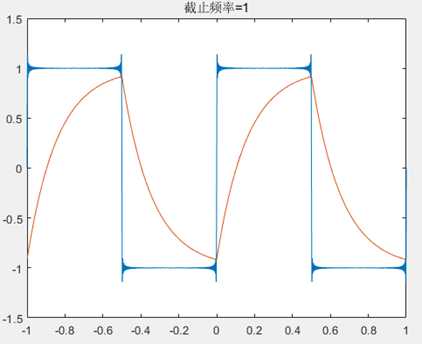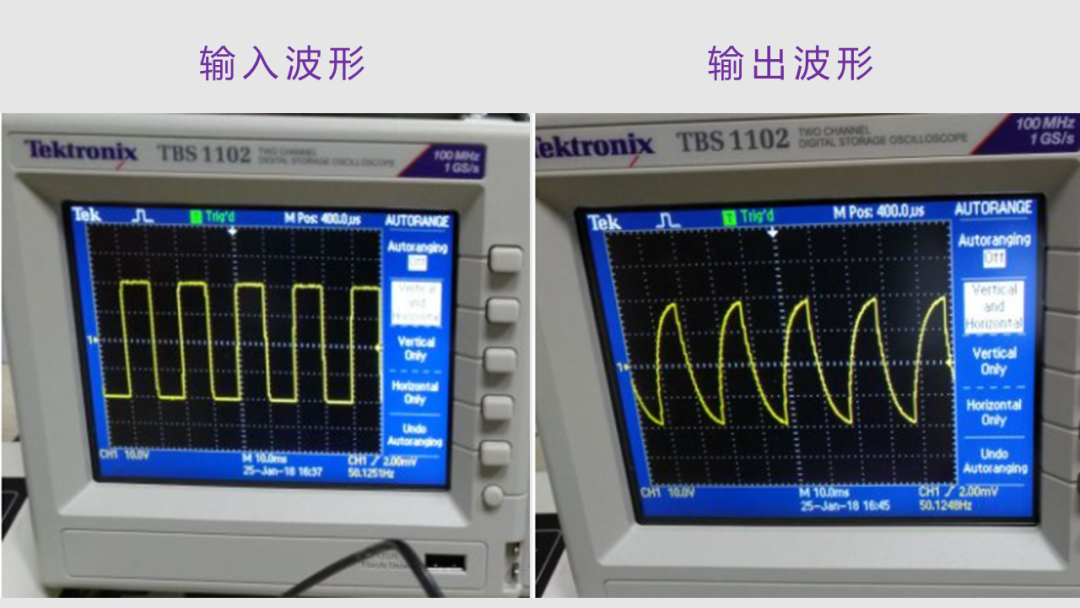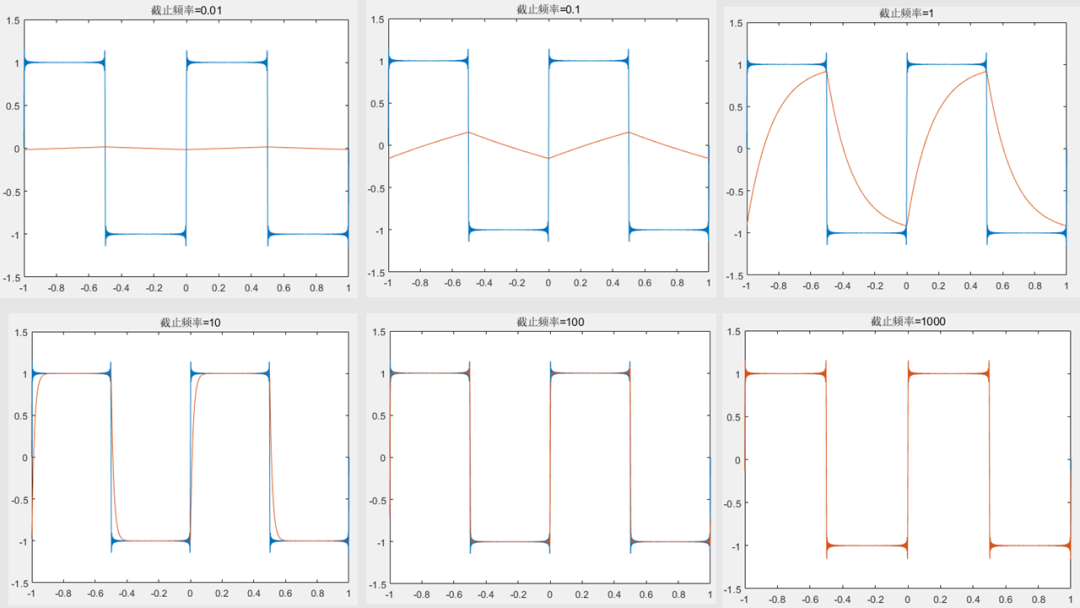0/200